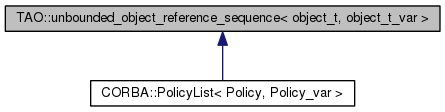TAO  2.0.8
TAO::unbounded_object_reference_sequence< object_t, object_t_var > Class Template Reference

`#include <Unbounded_Object_Reference_Sequence_T.h>`

Inheritance diagram for TAO::unbounded_object_reference_sequence< object_t, object_t_var >:[legend]

List of all members.

## Public Types

typedef object_t object_type
typedef object_typevalue_type
typedef value_type const const_value_type
typedef ::CORBA::ULong size_type
typedef
details::object_reference_traits
< object_type, object_t_var,
true >
element_traits
typedef
details::unbounded_reference_allocation_traits
< value_type, element_traits,
true >
allocation_traits
typedef
details::object_reference_sequence_element
< element_traits
element_type
typedef
details::object_reference_const_sequence_element
< element_traits
const_element_type
typedef element_type subscript_type
typedef value_type const & const_subscript_type
typedef
details::generic_sequence
< value_type,
allocation_traits,
element_traits
implementation_type

## Public Member Functions

unbounded_object_reference_sequence ()
unbounded_object_reference_sequence (CORBA::ULong maximum)
unbounded_object_reference_sequence (CORBA::ULong maximum, CORBA::ULong length, value_type *data, CORBA::Boolean release)
CORBA::ULong maximum () const
CORBA::Boolean release () const
CORBA::ULong length () const
void length (CORBA::ULong length)
const_element_type operator[] (CORBA::ULong i) const
element_type operator[] (CORBA::ULong i)
value_type const * get_buffer () const
void replace (CORBA::ULong maximum, CORBA::ULong length, value_type *data, CORBA::Boolean release=false)
value_typeget_buffer (CORBA::Boolean orphan=false)
void swap (unbounded_object_reference_sequence &rhs) throw ()

## Static Public Member Functions

static value_typeallocbuf (CORBA::ULong maximum)
static void freebuf (value_type *buffer)

## Private Attributes

implementation_type impl_

## Member Typedef Documentation

template<typename object_t, typename object_t_var>
 typedef details::unbounded_reference_allocation_traits TAO::unbounded_object_reference_sequence< object_t, object_t_var >::allocation_traits
template<typename object_t, typename object_t_var>
 typedef details::object_reference_const_sequence_element TAO::unbounded_object_reference_sequence< object_t, object_t_var >::const_element_type
template<typename object_t, typename object_t_var>
 typedef value_type const& TAO::unbounded_object_reference_sequence< object_t, object_t_var >::const_subscript_type
template<typename object_t, typename object_t_var>
 typedef value_type const TAO::unbounded_object_reference_sequence< object_t, object_t_var >::const_value_type
template<typename object_t, typename object_t_var>
 typedef details::object_reference_traits TAO::unbounded_object_reference_sequence< object_t, object_t_var >::element_traits
template<typename object_t, typename object_t_var>
 typedef details::object_reference_sequence_element TAO::unbounded_object_reference_sequence< object_t, object_t_var >::element_type
template<typename object_t, typename object_t_var>
 typedef details::generic_sequence TAO::unbounded_object_reference_sequence< object_t, object_t_var >::implementation_type
template<typename object_t, typename object_t_var>
 typedef object_t TAO::unbounded_object_reference_sequence< object_t, object_t_var >::object_type
template<typename object_t, typename object_t_var>
 typedef ::CORBA::ULong TAO::unbounded_object_reference_sequence< object_t, object_t_var >::size_type
template<typename object_t, typename object_t_var>
 typedef element_type TAO::unbounded_object_reference_sequence< object_t, object_t_var >::subscript_type
template<typename object_t, typename object_t_var>
 typedef object_type* TAO::unbounded_object_reference_sequence< object_t, object_t_var >::value_type

## Constructor & Destructor Documentation

template<typename object_t, typename object_t_var>
 TAO::unbounded_object_reference_sequence< object_t, object_t_var >::unbounded_object_reference_sequence ( ) ` [inline]`
template<typename object_t, typename object_t_var>
 TAO::unbounded_object_reference_sequence< object_t, object_t_var >::unbounded_object_reference_sequence ( CORBA::ULong maximum ) ` [inline, explicit]`
template<typename object_t, typename object_t_var>
 TAO::unbounded_object_reference_sequence< object_t, object_t_var >::unbounded_object_reference_sequence ( CORBA::ULong maximum, CORBA::ULong length, value_type * data, CORBA::Boolean release ) ` [inline]`

## Member Function Documentation

template<typename object_t, typename object_t_var>
 static value_type* TAO::unbounded_object_reference_sequence< object_t, object_t_var >::allocbuf ( CORBA::ULong maximum ) ` [inline, static]`
template<typename object_t, typename object_t_var>
 static void TAO::unbounded_object_reference_sequence< object_t, object_t_var >::freebuf ( value_type * buffer ) ` [inline, static]`
template<typename object_t, typename object_t_var>
 value_type const* TAO::unbounded_object_reference_sequence< object_t, object_t_var >::get_buffer ( ) const` [inline]`
template<typename object_t, typename object_t_var>
 value_type* TAO::unbounded_object_reference_sequence< object_t, object_t_var >::get_buffer ( CORBA::Boolean orphan = `false` ) ` [inline]`
template<typename object_t, typename object_t_var>
 CORBA::ULong TAO::unbounded_object_reference_sequence< object_t, object_t_var >::length ( ) const` [inline]`
template<typename object_t, typename object_t_var>
 void TAO::unbounded_object_reference_sequence< object_t, object_t_var >::length ( CORBA::ULong length ) ` [inline]`
template<typename object_t, typename object_t_var>
 CORBA::ULong TAO::unbounded_object_reference_sequence< object_t, object_t_var >::maximum ( ) const` [inline]`
template<typename object_t, typename object_t_var>
 const_element_type TAO::unbounded_object_reference_sequence< object_t, object_t_var >::operator[] ( CORBA::ULong i ) const` [inline]`
[]

template<typename object_t, typename object_t_var>
 element_type TAO::unbounded_object_reference_sequence< object_t, object_t_var >::operator[] ( CORBA::ULong i ) ` [inline]`
[]

template<typename object_t, typename object_t_var>
 CORBA::Boolean TAO::unbounded_object_reference_sequence< object_t, object_t_var >::release ( ) const` [inline]`
template<typename object_t, typename object_t_var>
 void TAO::unbounded_object_reference_sequence< object_t, object_t_var >::replace ( CORBA::ULong maximum, CORBA::ULong length, value_type * data, CORBA::Boolean release = `false` ) ` [inline]`
template<typename object_t, typename object_t_var>
 void TAO::unbounded_object_reference_sequence< object_t, object_t_var >::swap ( unbounded_object_reference_sequence< object_t, object_t_var > & rhs ) throw ()` [inline]`

## Member Data Documentation

template<typename object_t, typename object_t_var>
 implementation_type TAO::unbounded_object_reference_sequence< object_t, object_t_var >::impl_` [private]`

The documentation for this class was generated from the following file: